# Sphere Robot With Sox Controller In Different Terrains

## 1. Introduction

In our project we will simulate the movement of a sphere robot in the simulation package LPZ-Robots. We will take a closer look at the sphere robot with 3 masses which we control with the sox controller. The goal of our project is to analyze the behaviour of the robot in different terrains and see what movement patterns will emerge. In order to do so we will vary the geometric structure of the terrains and look at what happens if we change the learning parameter of the model and the controller(see section homeostasis/homeokinesis).
In the following we will explain how different terrains can be created within the simulation, how the controller works and discuss our results within the phase space of our system.

## 2. Setup

The buildup of the sphere robot with 3 masses can be seen in Fig. 2.1. It consists of a spherical outer shape, which contains 3 different rods within, each perpendicular to each other. On each rod one of the 3 masses is located whose movements will be determined by the implemented controller. Because we are trying to create self-organized behaviour the movement of the masses is not hard coded, rather it is determined by a process which takes into account the proprioceptive sensors and uses homeostasis and homeokinesis within a feedback loop to obtain semi-stable movement patterns.
This is where the sox controller plays a major role. It is a controller which implements homeokinesis in order to find new interesting behaviours.
The different terrains can be created within the LPZ simulation package by using the two classes playground and terrain. How to implement those can be seen in "Different Terrains".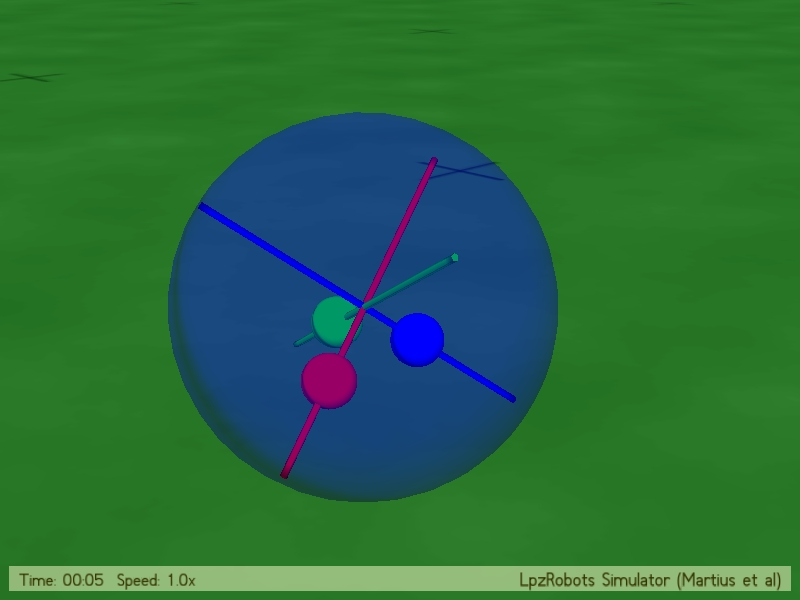Fig. 2.1: Sphere robot with three masses.

## 3. Different Terrains

As already mentioned the two classes needed to create terrains are playground and terrain. With the Playground the teal frame is created, while the class terrain is responsible for the 2 dimensional manifold on which the sphere will explore different movements. Furthermore the class terrain only allows us to create manifolds with elliptical curvature (see Fig 3.3, 3.4)
The shapes of our playground/terrains are hard coded, because we are not interested in movements that result form small changes to the shape. We took a look at it and saw that slight changes in the dimensions of the playground/terrains did not affect the movement of our robot in a significant way. Thus the user can choose at the start of the program between 4 options, whereby further can be added quickly.
The Playground is initialized by a pointer and then depending on which simulation we want to run a different case in the switch will be triggered, thus adjusting the size of the frame to the needed dimensions (see Fig. 3.1).
The Terrain is created in a very similar way, only the parameters needed for the functions are a little different (see Fig 3.2).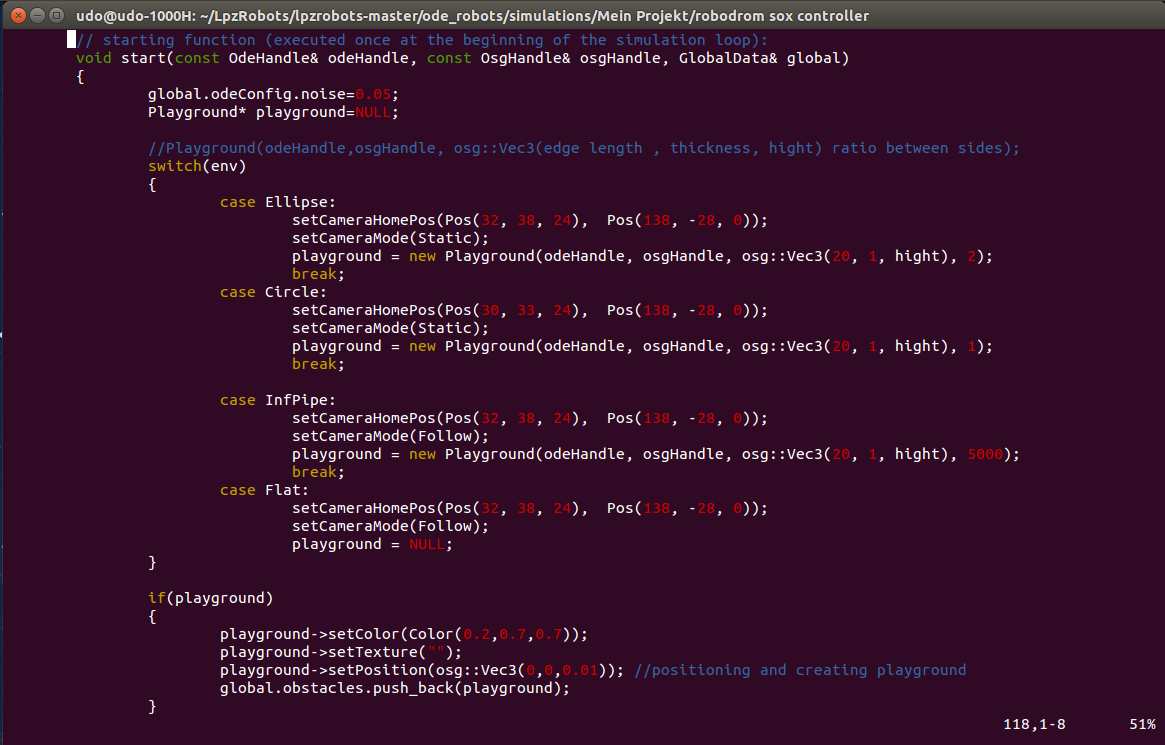Fig. 3.1: Playground Code. The command "new Playground(..., ..., osg::Vec3(edge length, thickness, height) ratio between sides)" sets the dimensions of the Playground, whereas the Parameters "edgelength, thickness, height, ratio between sides" must be set to the users desires. If the playground pointer is not NULL it will be colored and pushed back into the simulation.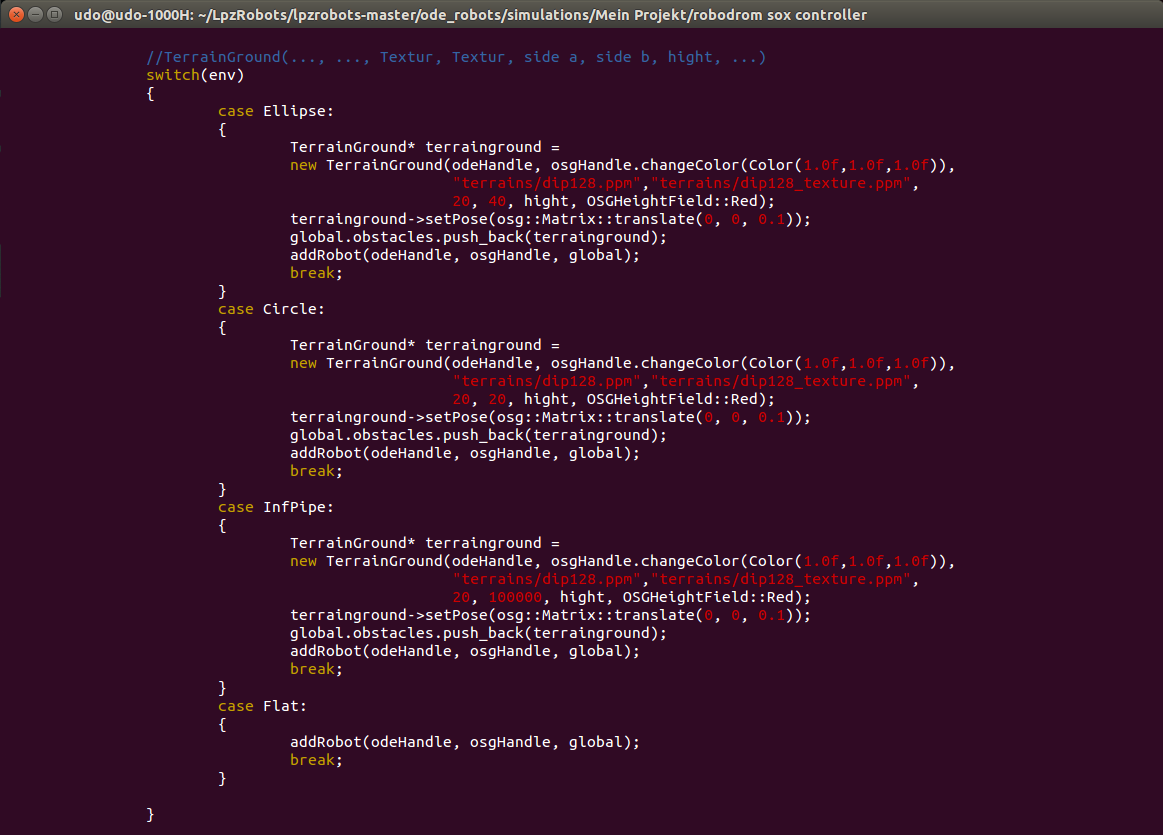Fig. 3.2: Terrain Code. The command "TerrainGround(..., ..., Texture1, Texture2, side a, side b, height, ...)" sets the dimensions of the Terrain, whereas the Parameters "side a, side b, height" must be set to the users desires. The Textures only serve an optical effect and do not have influence on the friction of the surface.

We took a closer look at 3 different general shapes: an endless pipe (very long ellipse), a spherical symmetry and an ellipse with ratio 2:1; Which can be seen in Fig 3.3.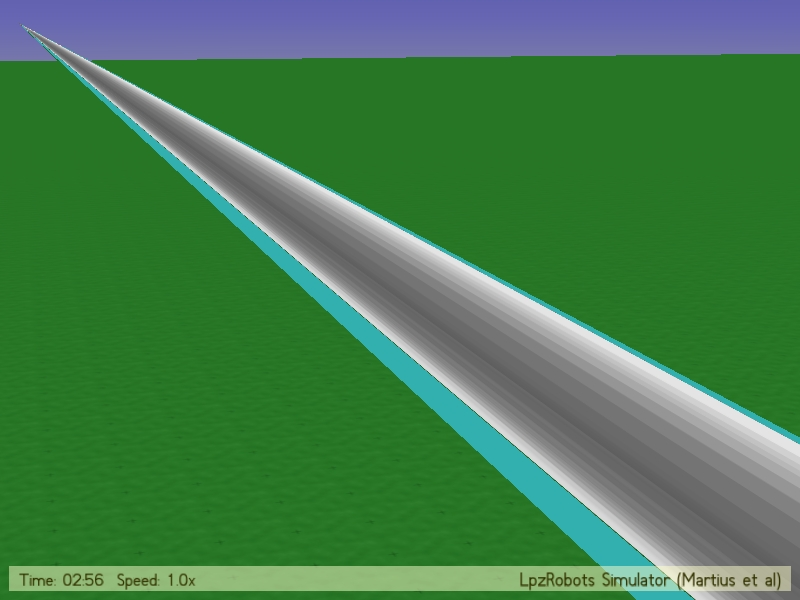Fig. 3.3: (a) Endless-pipe Terrain. (b) Spherical Terrain. (c) Elliptic Terrain. (click to enlarge)

Experimenting with the creation of different terrains, we also succeeded in constructing rather exceptional topologies. (To be clear, topologies is meant to be seen in a geographical way here.) We wanted to show some of them as an example in Fig. 3.4. Neither of these was further explored by in connection with the sphere robot. These were only built to test the implementation in a way.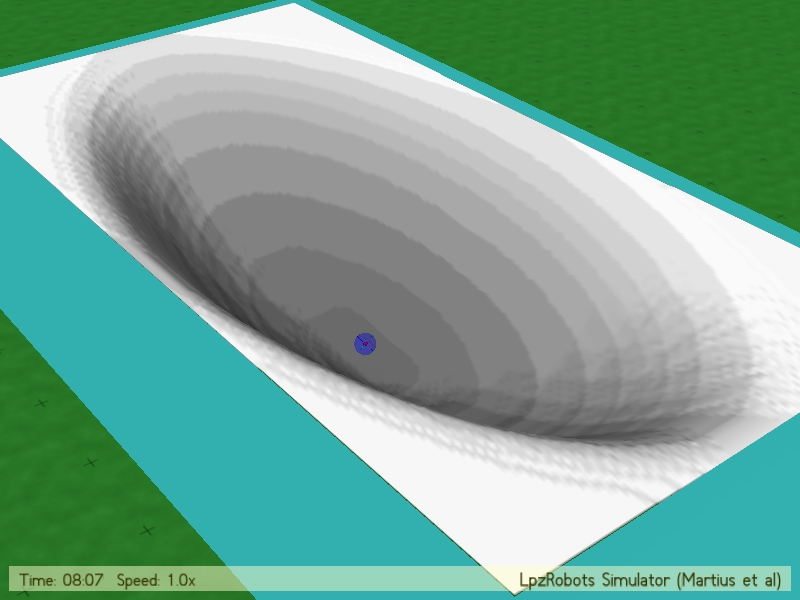Fig. 3.4: Several experiments with complicated Terrain-designs. (click to enlarge)

## 4. Sox-Controller

The sox-controller is a very advanced way of implementing the sensomotoric-loop (see below) in a self-organized robot. All credit for the implementation of this sophisticated algorithm goes to Martius and Der, who are also the foundation of the think-tank, that provided the LPZ-Robot-environment. The sox-algorithm is an explicit way of implementing homeokinesis. Before the exposition of the algorithm itself, a brief summary of the concept of homeokinesis is given.

### 4.1 Sensomotoric-Loop

To give a quick exposition of the nomenclature used in the following, it is useful to summarize the mathematical concept of the sensomotoric-loop.
In a given timestep:
\begin{align} \vec{x}_{t+1} = \vec{M}(x_t,y_t) + \vec{\zeta}_{t+1} \end{align} Where $\vec{x}_t$ is the sensor values and $\vec{y}_t$ is the motor values. The vector $\vec{M}$ gives the prediction of the sensor values in following timestep $\vec{x}_{t+1}$. Zeta, $\vec{\zeta}_{t+1}$ is a quantity to express the error of prediction.

As M can be expressed as $m \times n$-matrix A (where m is the number of motors and n is the number of sensors) and an independent bias vector $\vec{b}$: \begin{align} \vec{M}_{t} = \hat{A}(x_t,y_t) \cdot \vec{y}_t + \vec{b}_t \end{align} With this identity one can convert to: \begin{align} \vec{x}_{t+1} = \hat{A}(x_t,y_t) \cdot \vec{y}_t + \vec{b}_t + \vec{\zeta}_{t+1} \end{align}

### 4.2 Self-organisation

Homeokinesis is, as an extension to homeostasis, a model to provide self-organized learning. To distinguish between these two general principles and get a better understanding of homeokinesis, the concept of homeostatis will initially be described briefly.

#### 4.2.1 Homeostasis

The main interest of the conceptual founder of homeostasis, William John Ashby, was the ultrastability, which can be discovered in some biological systems. Originally Ashby wanted to built a machine, that could withstand heavy perturbations without loosing its intrinsic stability. He did so in the 1930s. The term homeostasis was coined by Cannon, a physiologist, in those days, too.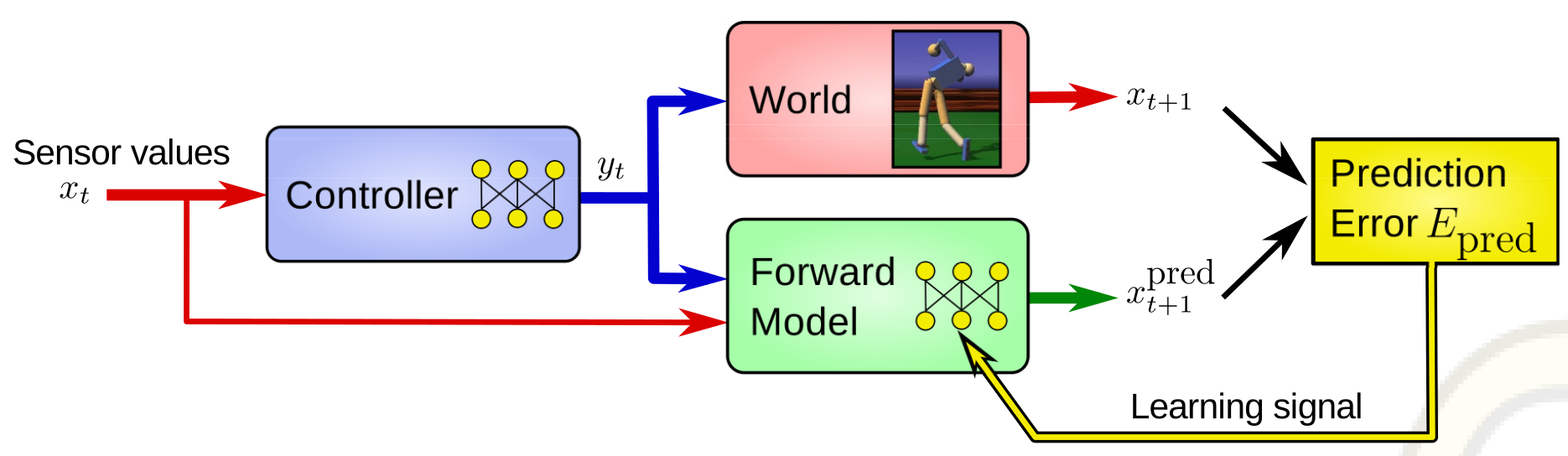Fig. 4.1:The sensorimotor loop and the forward model of the robot. The controller receives the current sensor values and generates corresponding motor values, which are sent both to the robot and its forward model. The difference between the predicted new senor values and the measured ones forms the prediction error $E_{pred}$. A learning signal for the improvement of the model is derived by gradient descending the error $E_{pred}$.  \begin{align} E_{pred} = \zeta^T\cdot \zeta \end{align} In intention to predict the values in the most exact way, which means minimizing the prediction error, homeostasis consequently compares predicted and actually measured sensor values. The resulting comparison can be expressed as model M with parameters a.
The change of these parameters is given as: \begin{align} \Delta a = - \epsilon_A \cdot \frac{\partial E_{pred}}{\partial a} \end{align} The variation of prediction matrix A and bias vector $\vec{b}$ can also be expressed as: $$\begin{eqnarray} \Delta A_{i,j} & = & \epsilon_A \cdot \zeta_i \cdot y_j\\ \Delta b_i & = & \epsilon_A \cdot \zeta_i \end{eqnarray}$$ The parameter $\epsilon_A$ is to be understood as the learning rate of the model. Which is one of the major subject of our project.

#### 4.2.2 Homeokinesis

Expanding the homeostatic concept, homeokinesis provides an opportunity for the system to explore certain semi-stable states by itself, where homeostasis was designed to stay in a given state in an ultrastable manner.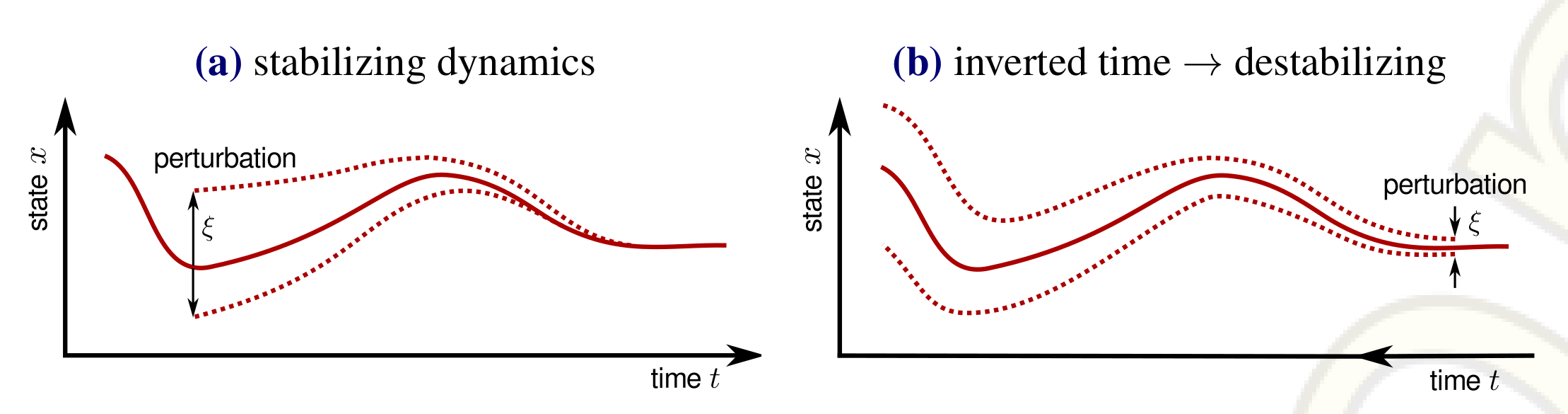Fig. 4.2:Inversion of time converts stability into instability. (a) Schematic evolution of a state and its perturbations in a stabilizing dynamics, approaching an attractor. (b) If the arrow of time is inverted, the attractor is converted into a repeller and small perturbations are amplified. In homeokinesis, a controller learns to stabilize a dynamics backward in time. If applied to the real system (that is running forward in time), the controller aims at destabilizing the dynamics.

"While homeostasis is seen as a general paradigm for the self-regulation towards stability, homeokinesis is intended as its dynamical counterpart - a general principle that drives agents to activity and innovation." 
The new essential quantity for the concept of homeokinesis in comparison to homeostasis is the Time Loop Error (TLE).
In the following mathematical approach, the vector-arrows will be dropped. Substitution:

\begin{align} \Psi(x_t) = M(x_t,K(x_t)) \end{align} \begin{align} x_{t+1} = \Psi(x_t) + \zeta_{t+1} = \Psi(x_t + v_t) \end{align} Introducing a variable $v_t$ that indicates an input-shift to reconstruct the true input ($x_t$) from the observed input ($x_{t+1}$) . To rephrase this transformation:
One switches from assuming a biased prediction to assuming a sensory bias.
Because we want to reconstruct the sensor values $x_t$ from the next values $x_{t+1}$ this becomes a nonlinear inverse problem. \begin{align} x_t +v_t = \Psi(x_{t+1})^{-1} \end{align} We define $v'_t$ as the input shift such that fulfills the equation: \begin{align} v'_{t} = arg min ||x_{t+1} - \Psi(x_t + v_t)|| \end{align} Hence we can calculate the Time-Loop Error ($E$): \begin{align} E = {v'}^T_t \cdot v'_t \end{align} The latter is also referenced as reconstruction error :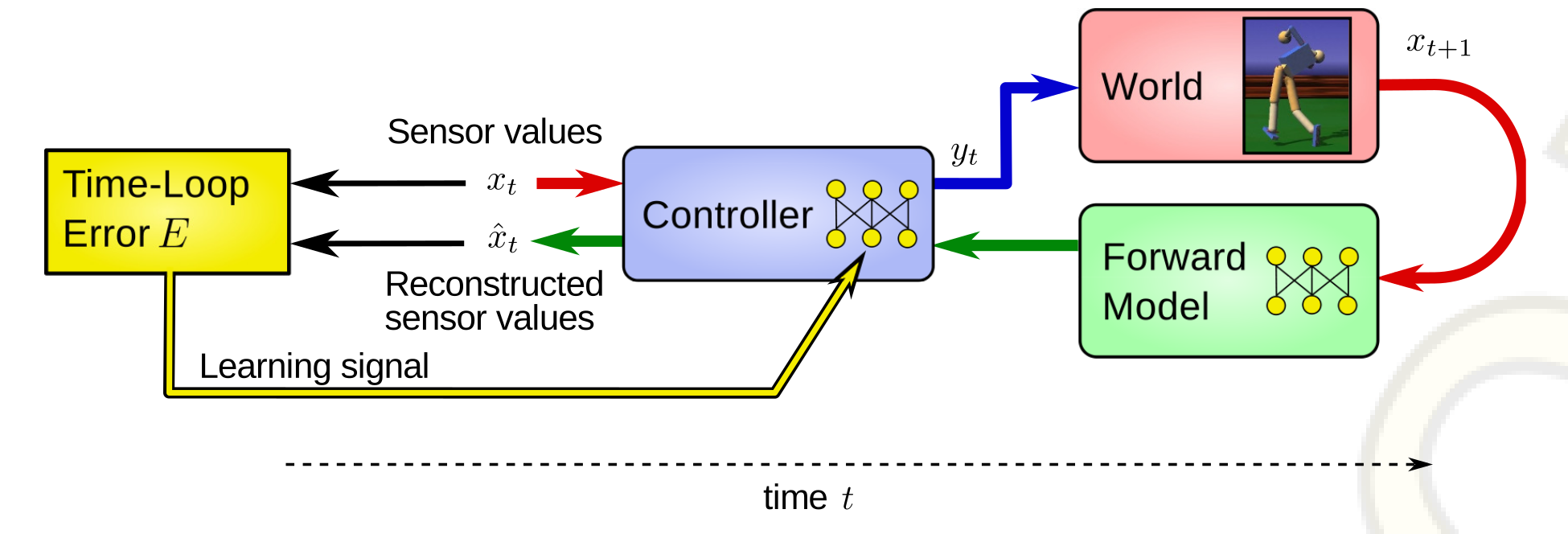Fig. 4.3: Inversion of time converts stability into instability. Starting at time t with sensor vector x, the signal flow in the time loop runs through the controller and the world (robot) producing the new sensor values at time t+1, which are projected backward through the forward model and the controller to yield the reconstructed sensor values $\hat{x}_t$. The difference between reconstructed and true sensor values forms the time-loop error (or reconstruction error), which is used as a learning signal for the controller. Following the application of the Taylor-Expansion and several transformations, one gets an identity which can be worked with: \begin{align} E = {v'}^T_t \cdot v'_t = \zeta^T \left(L L^T\right)^{-1} \zeta \end{align} Where L is the Jacobian-matrix of $\Psi$ $$\begin{eqnarray} \hat{L}(x) &=& \frac{\partial \Psi(x)}{\partial x} \\ L_{ij}(x) &=& \frac{\partial \Psi_i(x)}{\partial x_j} \end{eqnarray}$$
##### learning controller

In addition to the learning rate of the model ($\epsilon_A$) a learning rate of the controller ($\epsilon_C$) can be introduced. This happens in a similar manner. In contrast to the the parameters a, which characterized our model-matrix A, for the derivation of $\epsilon_C$ parameters p are used, which characterize the controller. Their change is derived as follows: \begin{align} \Delta p = p_{t+1} - p_t = \epsilon_C \cdot \frac{\partial E}{\partial p} = \epsilon_C \cdot \chi^T \cdot \frac{\partial L}{\partial p } \cdot v \end{align} Where $\chi$ is a substitution for: \begin{align} \chi = \left(L^T\right)^{-1} \cdot v \end{align} The parameter $\epsilon_C$ is to be understood as the learning rate of the controller. Which is one of the major subjects of our project.

### 4.3 Implementation

The concrete implementation of the sox-algorithm for the LPZ-Robots-environment is a quite sophisticated and extensive piece of programming work. The credit for this goes completely to the inventors and founders of LPZ and especially to Ralf Der and Georg Martius. In the following we give a short overview of the implementation. In doing so, we focus on the quantities of $\epsilon_A$ and $\epsilon_C$, since these were the parameters whose general effect on the robot we were interested in.
So far we have explained how homeostasis and homekinesis work, but only in general theoretical terms. Now we want to explain how the learning rules can be implemented in such a way that they are easier to code. In order to make the calculations simpler we introduce 5 vectors - respectively matrices, of which some are already known. First of all we have the parameters of the controller ($C,h$). We use a one-layer neural network for our controller, which we call $K(x)$: \begin{align} K(x) = g(C \cdot x+h) \end{align} Thus $C$ is a $m \times n$ synaptic matrix, $h$ an $m$-dimensional bias vector and g the activation function, which in our project is implemented as $tanh$ (used to map the values smoothly to the interval [-1,1]). One has to mention that g($\vec{\alpha}$) in our notation means that we apply the function g to each value of the vector separately. The update rules for the parameters of the controller ($C,h$) are given by the gradient descent of the TLE: \begin{align} \Delta C = \epsilon_C \mu v'^T - \epsilon y x^T \end{align} \begin{align} \Delta h = -\epsilon y \end{align} With $\mu$ = G'A^T$${L^{-1}}^Tv and G'=g\cdot \underline{\mathbb{1}} because we use the same activation function for every sensor. Furthermore \epsilon is a diagonal matrix with \epsilon_{ij} = 2\epsilon_C$$\alpha$$\delta_{ij}$$\mu_i$$\zeta_i. Especially one can see that the change of the matrices A and C are connected via \mu. This has the effect that \epsilon_A and \epsilon_C must both be bigger then 0, otherwise the controller is incapable of learning. The other 3 important factors are the parameters of the forward model (A,S,b), which are obtained by gradient descending the prediction error E^{pred} = \zeta^T \zeta. With the forward Model M:$$\begin{align} M(x,y) = Ay + Sx + b \end{align}$$A being a m\times n matrix, representing the response of the sensors to the motor values, b the modeling error vector and S being an m \times m matrix, an extension to the earlier forward model, including the sensor-to-sensor mapping. The update rules for these parameters are as follows:$$\begin{align} \Delta A = \epsilon_A \xi y^T \end{align}\begin{align} \Delta b = \epsilon_A \xi \end{align}\begin{align} \Delta S = \epsilon_A \xi x^T \end{align}$$At the beginning of a simulation with the sox-controller all matrices are set to unit matrices and the function sox::step is called. This function (Fig. 4.4) is used in each iteration of the simulation. It does a step by calling the function stepNolearning (Fig. 4.4) which simply uses the given matrices to send the next motor signal. In the same iteration step the function learn is called, which includes the whole implementation of the theory mentioned above, thus changing the matrices A,C,S and the vectors h,b. This way the controller learns how to use homeokinesis to break free of repetitive patterns.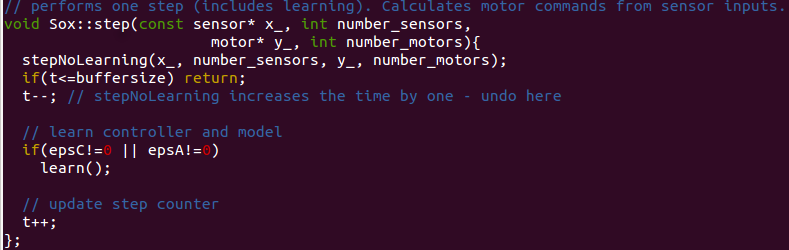Fig 4.4: Sox::Step. Function, that is called every simulation timestep. It furthermore calls a function to send upcoming motor signals, as well as a function to update the learning parameters, if applicable (function learn)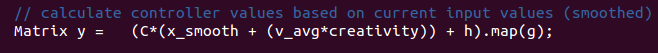Fig 4.5: Calculation of motor values. Central process in function stepNoLearning: Here the motor signals are calculated. To summerize exactly what the outputs of the sox-controller to the motors are one can look at the following equation, whose implementation can be seen in Fig. 4.5:$$\begin{align} y_j = tanh\left[\sum_i C_{j,i} \cdot \left(v_{avg}\cdot \beta + x_{smooth,i} \right) +h_j \right] = tanh \left[ \sum_i C_{j,i} \cdot x_{i} +h_j \right] \end{align}y_j$is the motor value that is send to motor j, tanh is used to map the values on [-1,1], because we want to limit the motor values.$C_{j,i}$is the matrix in which all the learning parameters have their contribution and which does the actual learning. (Note: although not denoted explicitly the three parameters$A,S,b$affect this term implicitly as well).$\beta$is the so called creativity, which we haven't explained, because it is set to zero in our simulation, thus the equation simplifies. The parameter$h_j$is one component of our bias vector h mentioned above and the last variable$x_{smooth,i}\$ is the average over the last few sensor values of sensor i. How many values are used for averaging can be set with the variable steps4Averaging, which is set to 1 in our simulation, meaning that we don't average at all and only use the last measurement.

Last it needs to be mentioned that the damping-item was also left as the default value of 0.00001. This quantity can be understood as a quantification of the forgetfulness of the model.
The implementation of the sox-controller provided the possibility for changing most of these mentioned values live during the simulation. We chose to set these values as a console input at the beginning of the simulation, because in that way, it was easily comprehensible in the logging file, with which parameter set the simulations had been run. We logged the controller parameters as well as the positions of the robot and calculated velocities from there with a python script manually (in cases, where the trajectory was interesting for us).

## 5. Observed pattern

In the following we would like to show some behavioural patterns we observed in different terrains. These patterns are of different complexity which is mainly because of varying the values for epsA and epsC. The model can be drastically simplified by setting these values to zero, which was our first approach to get a good feeling for the controller. Showing the patterns in order of increasing abilities of the controller we would like to clearify a bit what capabilities these quantities epsA and epsC provide the robot with.

### 5.1 pipe

As a first example we chose the terrain with the most simple symmetry. Additionally we set epsA and epsC to zero. The result is a rather trivial behaviour, but it clarifies an important fact about the controller. By choosing these parameters we effectively deactivated the learning ability of the controller. The result is an anyhow very stable moving pattern. The homogeneity of the resulting motion is analyzed in the following section. Observing this pattern by eye one already identifies the stable limit cycle.

### 5.2 sphere

Our Investigation of the sphere robot in a spherical basin provided very similar but in detail still diverse behaviours. It is no big surprise, that the robot achieves patterns in a circular symmetrical track, due to the intrinsic symmetry of the terrain. In this setup it is rather apparent to see what an increment of epsC causes.

#### 5.2.1 stable circle

In this first approach again epsA was set to 0.0 and epsC was set to 0.0. We observed a very stable circular movement. the radius of this movement pattern was constant for a relatively long period of time (see following section for further analysis)

#### 5.2.2 Unstable Circle

By increasing epsC to 0.3 as well as setting epsA to 0.3, one observes circular motion of quite variable radii. In a way this is not extremely surprising, since we increased the learning rate, and by that gave the controller the ability to explore. We also tried increasing the learning rate in this terrain to a rather extensive amount and observed an amusingly explorational behaviour of the robot, that can be watched at the bottom of this page.

### 5.3 Elliptic Basin

With a disturbance of the spherical symmetry by shifting to an elliptical basin, the limit-cycles of circular moving pattern destabilize. The analysis of the resulting movement becomes very complex due to lack of stability as well as complexity of the motion itself. Observing these pattern by eye, a periodical motive can be discovered, but it is not really consistent and additionally breaks down frequently.
This Simulation was performed with parameter-values of epsA = 1.0 (which is supposed to be the maximum of reasonably chosen values) and epsC=0.0. In this configuration the robot already seems to be very eager to try out new things, which is an actual fallacy. Taking a closer look at the implementation, as mentioned epsA does not have an practical effect on learning if epsC is set to zero. As a result all discovered moving pattern are destabilized fast and in a way disregarded by the controller.

### 5.4 Flat Terrain

As a last experiment we wanted to investigate the behaviour of the sphere robot - respectively, the sox-controller - with a somehow pathological configuration. We set the learning rate of the controller (epsC) to 10, which is much higher then reasonable (which would be between 0 and 1). By this we wanted to get a picture what a pathologically high learning rate could be. (We were just curious...)
The observed movements exhibit a kind of repeating pattern, which is in no way harmonic. Even though the robot was nominally learning in a high rate, it hardly ever left this movement motive. We performed this simulation in a completely flat terrain to be sure, that the obtained sensor values only appeared from the motions immanently performed by the controller.

## 6. Analysis

In the following section two movement pattern will be analyzed regarding their harmonicity. Therefore the phase space is observed. In this manner we would like to reveal the limit-cycles the controller reaches. Initially the raw data output will be shown, before the pattern will be extracted from the phase space.

### 6.1 Sinus

As a first example we would like to take a closer look at the resulting phase space of the sinusoidal motion of the sphere robot in the endless pipe terrain. An excerpt of the raw data can be observed in Fig. 6.1.1. As an observable for the position in space, we introduce the distance of the robot to the axis of the pipe. After a short phase of initiation, a relatively constant amplitude of the oscillating motion can be observed. The starting phase is also interesting to be investigated in the graphs of velocities. The velocity in y-direction, which is the direction the pipe expands is a quite demonstrative quantity to estimate the noise.
Since there is no relevant motion in z-direction (which is the direction of the sky), this coordinate is neglected in further analysis (as well in the subsequent section). The resulting phase space is naturally very crowded and looks more like chaos, although there is a similarity to phase space trajectories of a simple harmonic oscillator.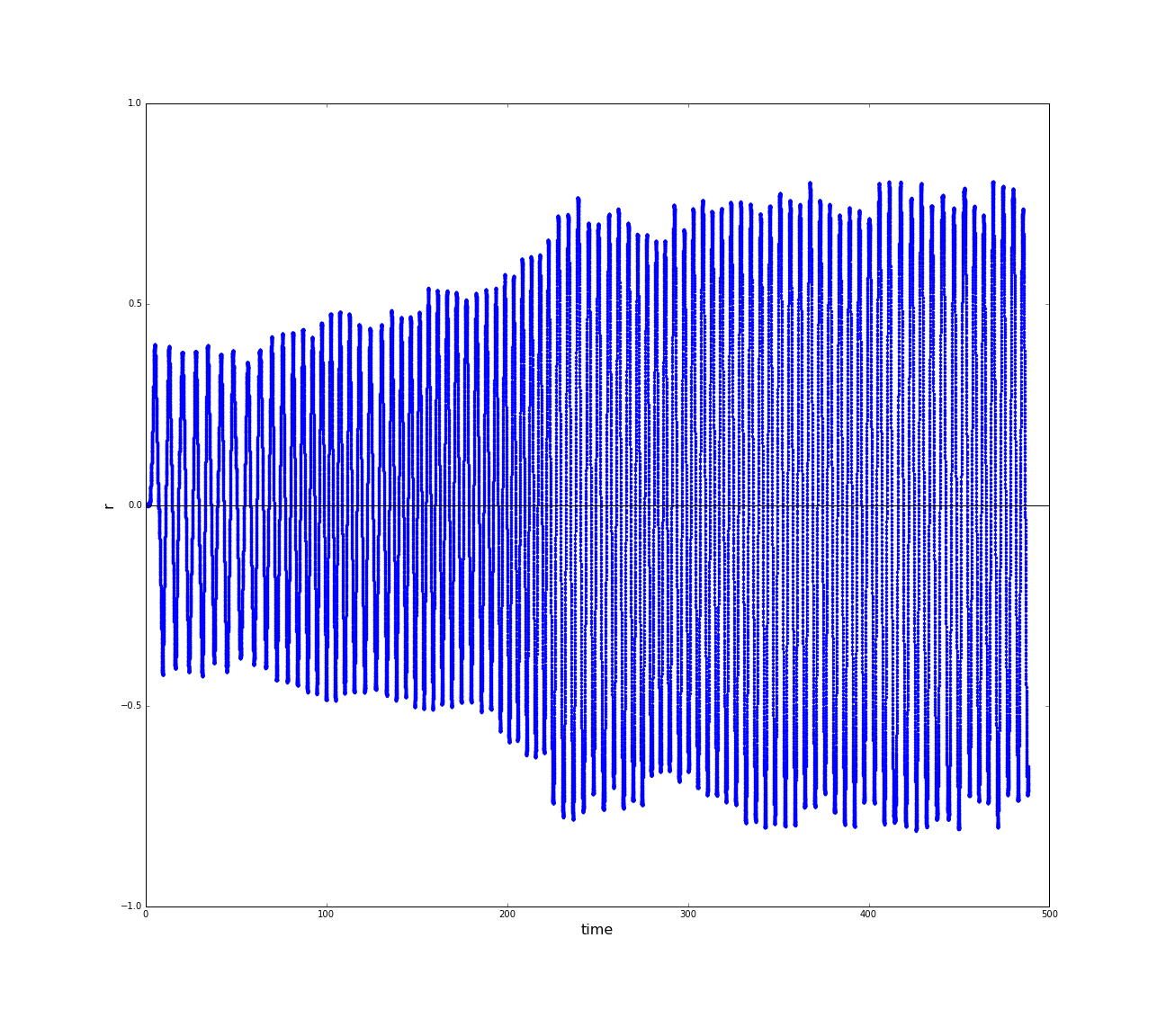Fig. 6.1.1: Raw data of sinusoidal movement pattern (a) Distances of the sphere robot to the expansion-axis of the pipe; (b) Velocities of the sphere robot in cartesian x,y and z-direction; (c) Phase spaces of the motion of the sphere robot in x and y direction. (click to enlarge)

Since the observed movement was expected to be oscillating perpendicular to the cylinder axis of the pipe we furthermore excerpted the x-positions and -velocities of a period in the trajectory, that was clearly decorrelated to the starting phase (t=[300,400]) and reviewed the phase space in this period. Since the motion in z-direction was zero in a good approximation, we approached x as equal to the distance to the propagation-axis.
The result is a limit-cycle with moderate noise. Certainly this outcome is no big surprise and in a way trivial, but again one has to keep in mind how stable and reproducible this motion pattern is achieved. See that the given system of the sphere robot is already quite complex (imagine to steer the motors yourself...).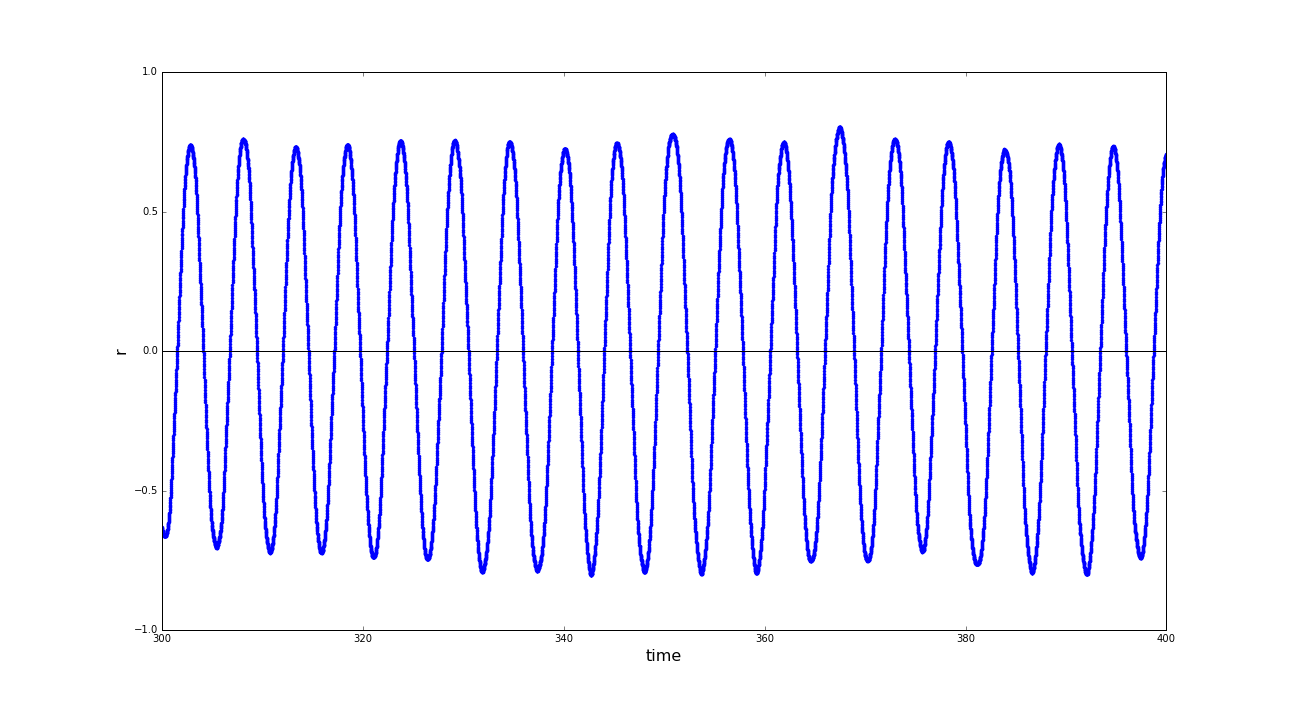Fig. 6.1.2: Excerpt of sinusoidal movement pattern in endless pipe environment (a) Distances of the sphere robot to the expansion-axis of the pipe from t=[300,400]; (b) Phase space of the motion of the sphere robot in x direction. (click to enlarge)

### 6.2 Stable Circle

The second example of motion patterns observed with the sphere robot is a little more complex then the first given example. Firstly the expected limit-cycle is located in two-dimensions and additionally it is not as stable as the precedingly described sinusoidal moving pattern.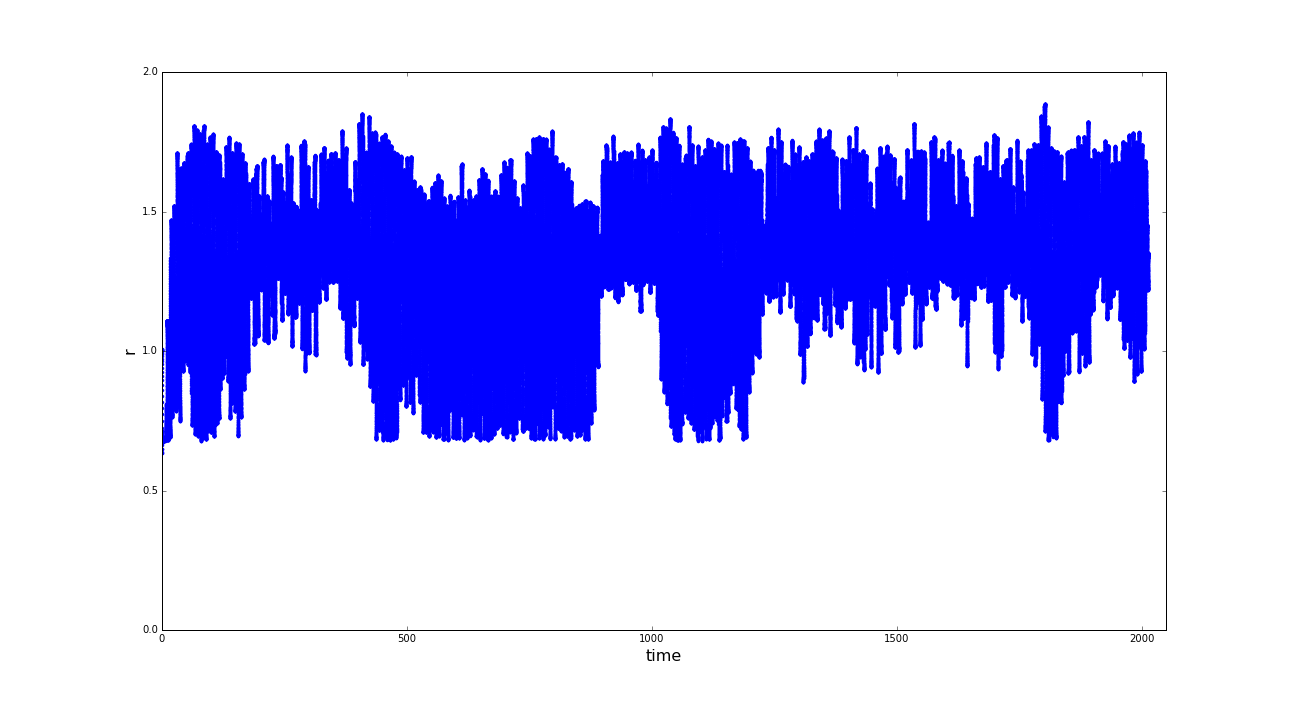Fig. 6.2.1: Raw data of circular movement pattern in spherical basin (a) Distances of the sphere robot to the center of the spherical basin; (b) Velocities of the sphere robot in cartesian x- and y-direction; (c) Positions of the sphere robot in a coordinate system rooted in the center of the spherical basin. (click to enlarge)

A first look at the raw data looks rather like chaos. As an approach to reduce the complexity of the problem we introduce the distance to the center of the basin as a new obervable. On whose plot the instability of the spherical motion can be observed, as well as on the remaining raw data. In the following the z-coordinate again be neglected in the Analyzation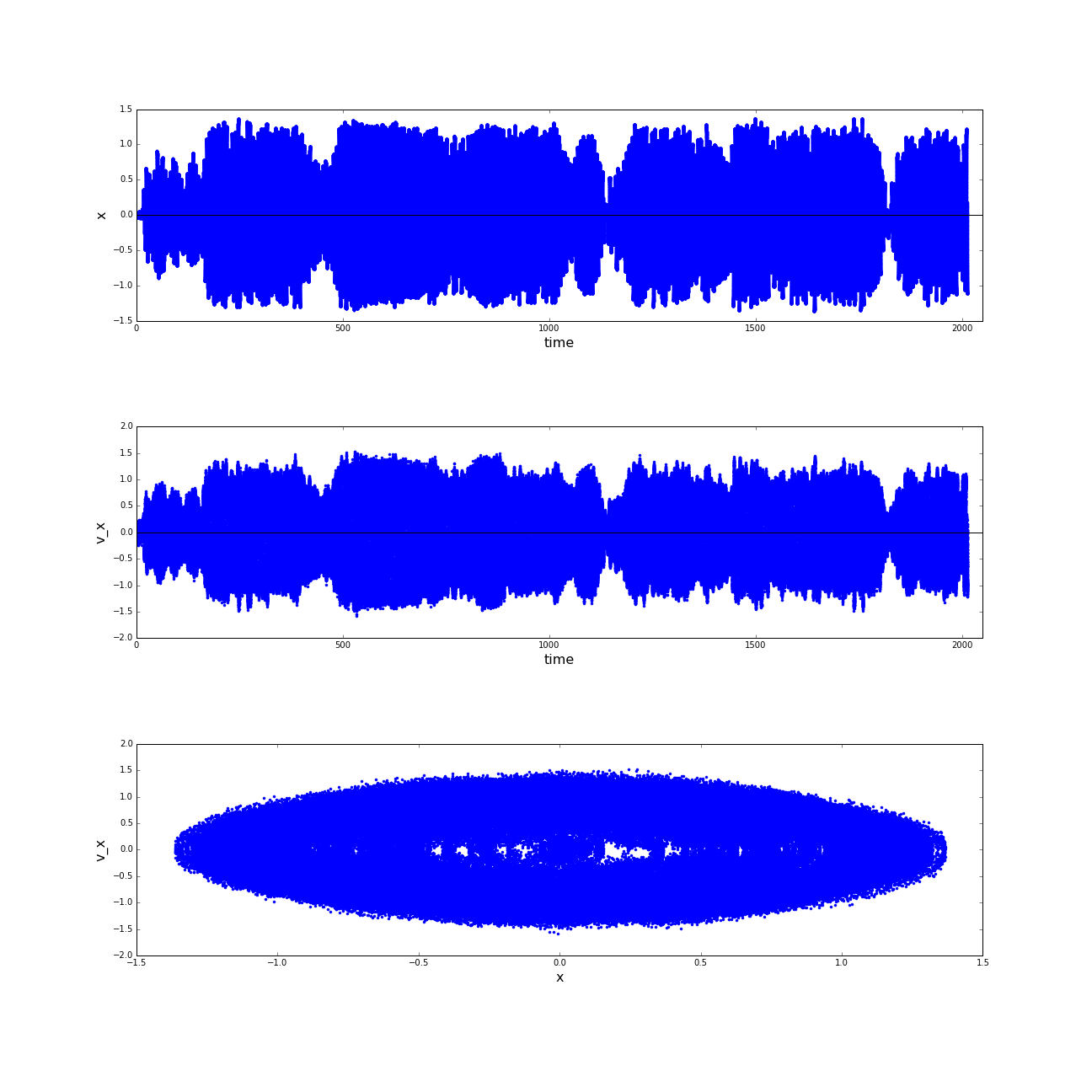Fig. 6.2.2: Phase space diagrams of the motion in spherical basin (a) Phase spaces of the motion of the sphere robot in x direction; (b) Phase spaces of the motion of the sphere robot in y direction. (click to enlarge)

Regarding the phase spaces of the motion in x and y direction separately, there is still only a meager hunch of an oscillating motion. Nevertheless a dominant state can be observed in these diagrams. The condition with big amplitudes of motion is comparably stable, whereas the conditions with lower distances to the center of the basin are in no way preserved.Fig. 6.2.3: Transforming coordinate-system to movement-center (a) Distances of the sphere robot to the center of the spherical basin in the time interval t=[900:1000] before shifting to coordinate system; (b) Positions of the sphere robot on the y-axis, before and after the shifting of the coordinate system; (c) Distances of the sphere robot to the center of the spherical basin in the time interval t=[900:1000] after shifting to coordinate system. (click to enlarge)

A very contra intuitive fact, that crossed our way, that still has not been explained to us is, that the sphere does not circle around the exact center of the basin. Observing the distance of the sphere to the center of the basin (r) in a rather constant region of the trajectory we still detected unexpectedly big variances. Taking a closer look at the positions in y direction we found an offset in the oscillation-pattern, which then was shifted back (in a mathematical way, we changed to a displaced coordinate system). Reviewing r after this adjustment we observed a much more stable condition.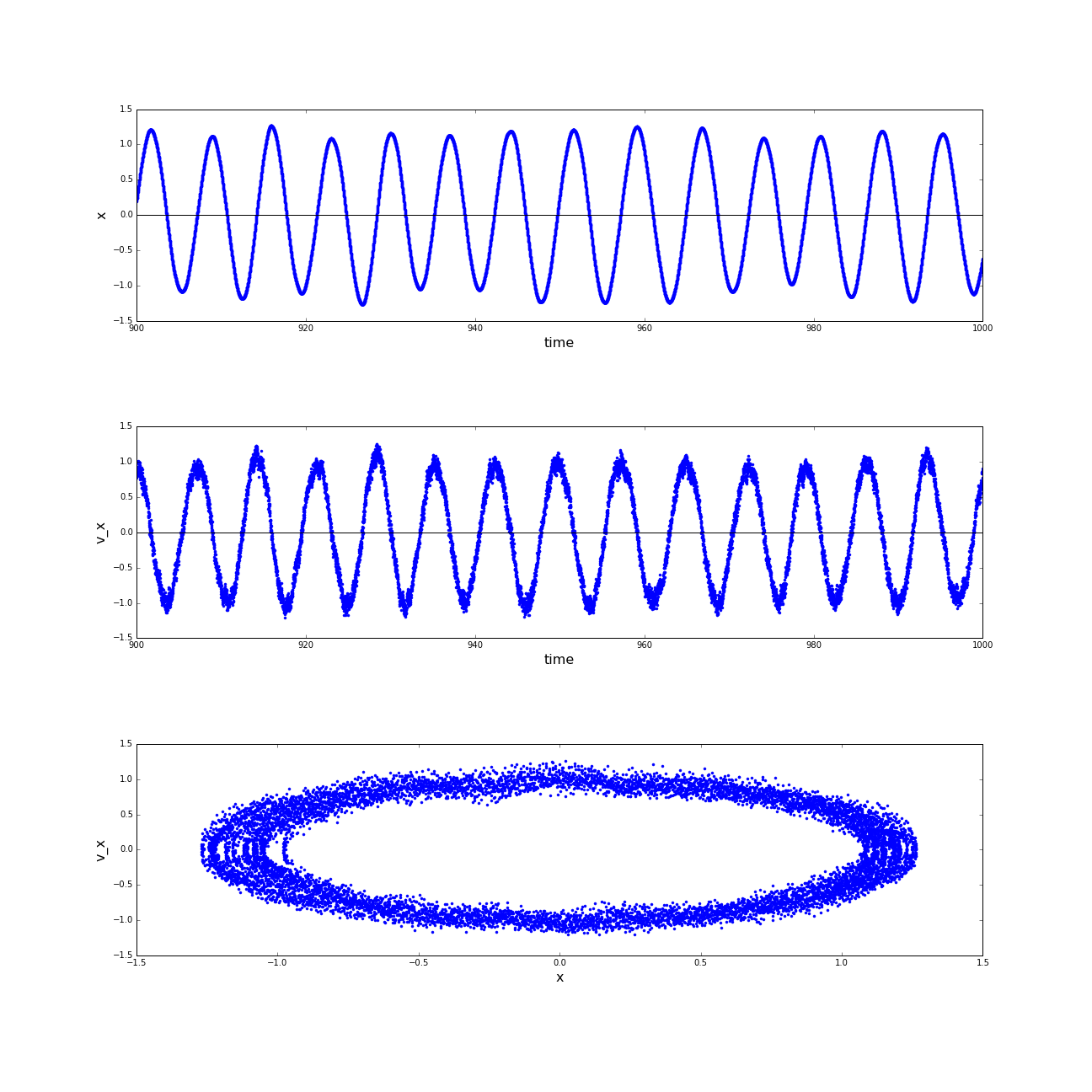Fig. 6.2.4: Resulting limit-cycle in Phase space (a) Phase spaces of the motion of the sphere robot in x direction in time interval t=[900:1000]after shifting the coordinate system; (b) Phase spaces of the motion of the sphere robot in y direction in time interval t=[900:1000]after shifting the coordinate system. (click to enlarge)

Again we can constitute the excerpts of the trajectory in phase space and get a 2-Dimensional oscillating motion as a result. The observed limit-cycles are only moderately noisy and were at least stable for 100 s of simulation time.

## 7. Conclusion

The sox-controller is even with some major simplifications, as for example setting the creativity to zero, still a complex way of implementing self-organization to a simulation environment. With its powerfulness comes also the property, that it only be predicted in a limited way. Of course that is also an ability, that is appreciated of a learning system in a way. This makes the analysis even more tricky, than it is anyways.
A clear outcome of our project is that some attractors in phase space, which are given by the natural consistency of the terrains are very strong. These certainly depend on the resulting potential that emerges due to the terrain topology (which is meant in a geographical context here). Depending on the learning rate of the robot it can overcome these heavy attractors. This is the explorational property, that homeokinesis is supposed to provide. All this is implemented in the SOX-Controller and can manifoldly be experimented with.